# MP Board Class 7th Maths Solutions Chapter 15 Visualising Solid Shapes Ex 15.2

## MP Board Class 7th Maths Solutions Chapter 15 Visualising Solid Shapes Ex 15.2

Question 1.
Use isometric dot paper and make an isometric sketch for each one of the given shapes: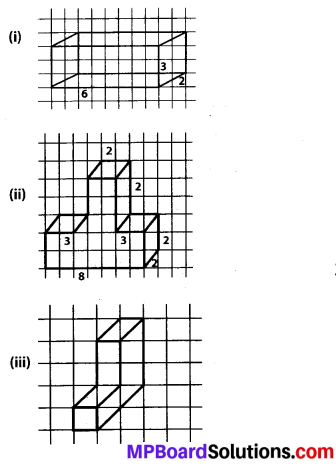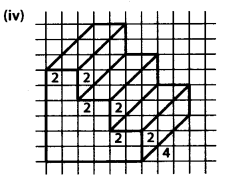Solution: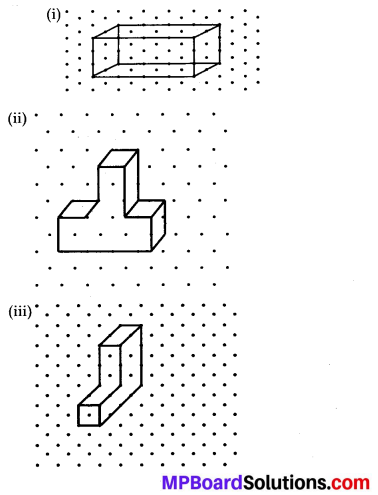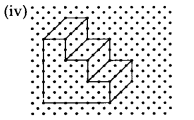Question 2.
The dimensions of a cuboid are 5 cm, 3 cm and 2 cm. Draw three different isometric sketches of this cuboid.
Solution:
3 isometric sketches of the given cuboid can be drawn as follows.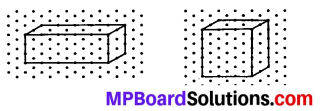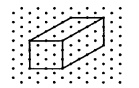Question 3.
Three cubes each with 2 cm edge are placed side by side to form a cuboid. Sketch an oblique or isometric sketch of this cuboid.
Solution:
When three cubes, each of 2 cm edge, are placed side by side, a cuboid with dimensions as 6 cm, 2 cm and 2 cm will be formed.Question 4.
Make an oblique sketch for each one of the given isometric shapes: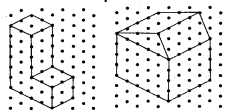Solution:
The oblique sketch of these figures will be as follows.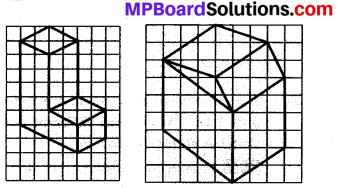Question 5.
Give (i) an oblique sketch and (ii) an isometric
sketch for each of the following:
(a) A cuboid of dimensions 5 cm, 3 cm and 2 cm. (Is your sketch unique?)
(b) A cube with an edge 4 cm long.
Solution:
(i) Oblique sketch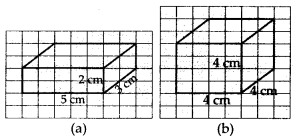(ii) Isometric sketch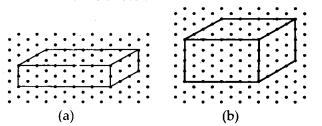The sketch for cuboid is not unique. The cuboid can also be drawn by taking the length as 3 cm or 2 cm. This will lead to a different view of the same cuboid.